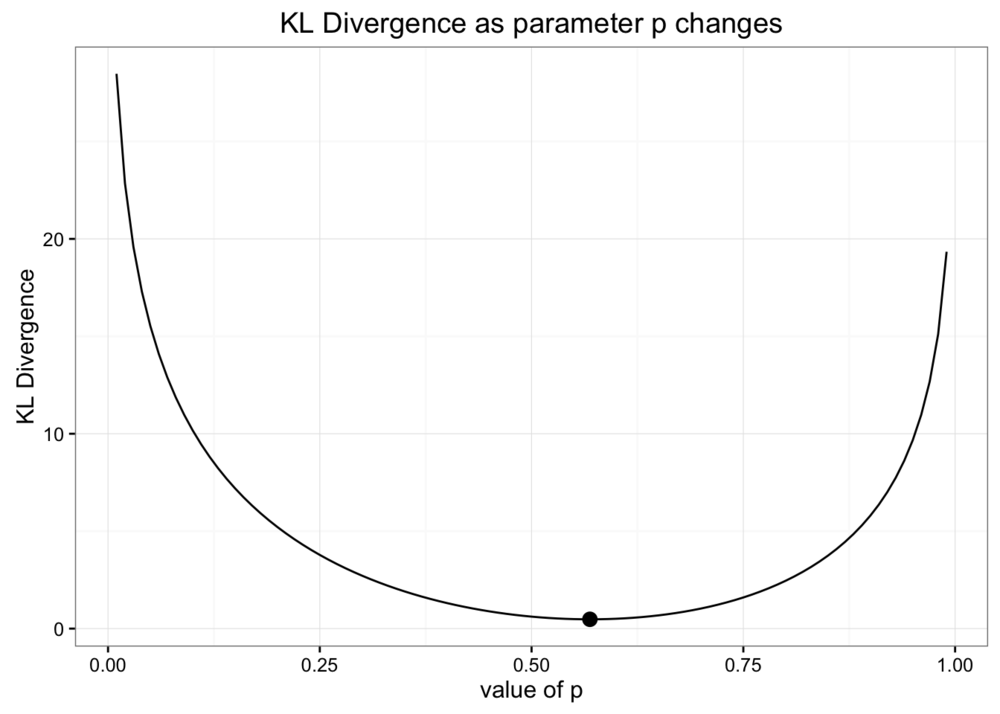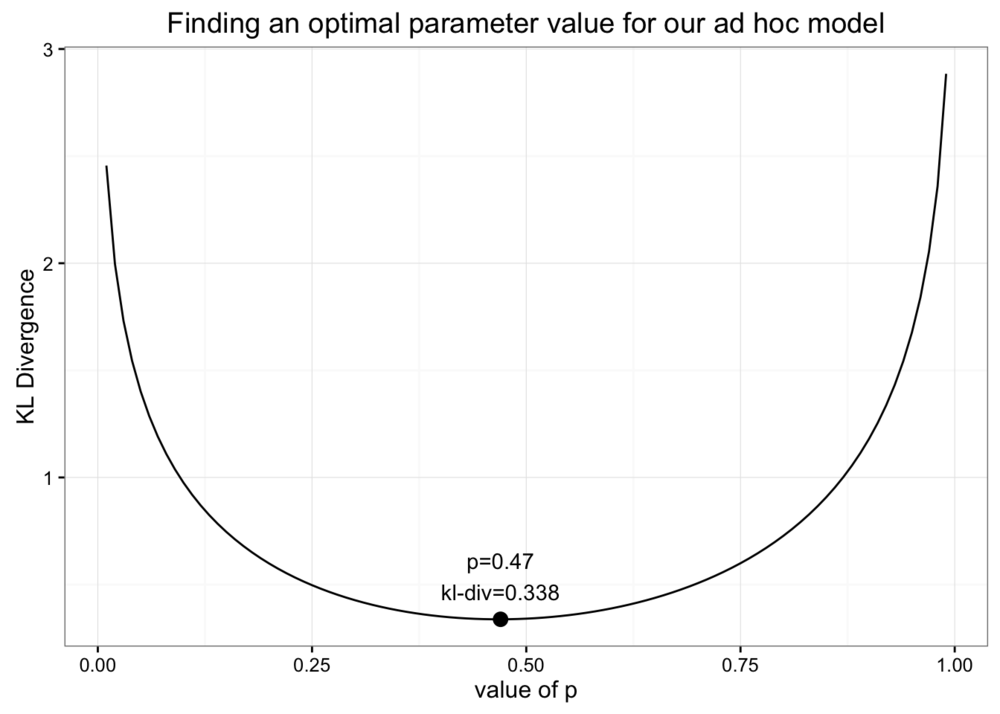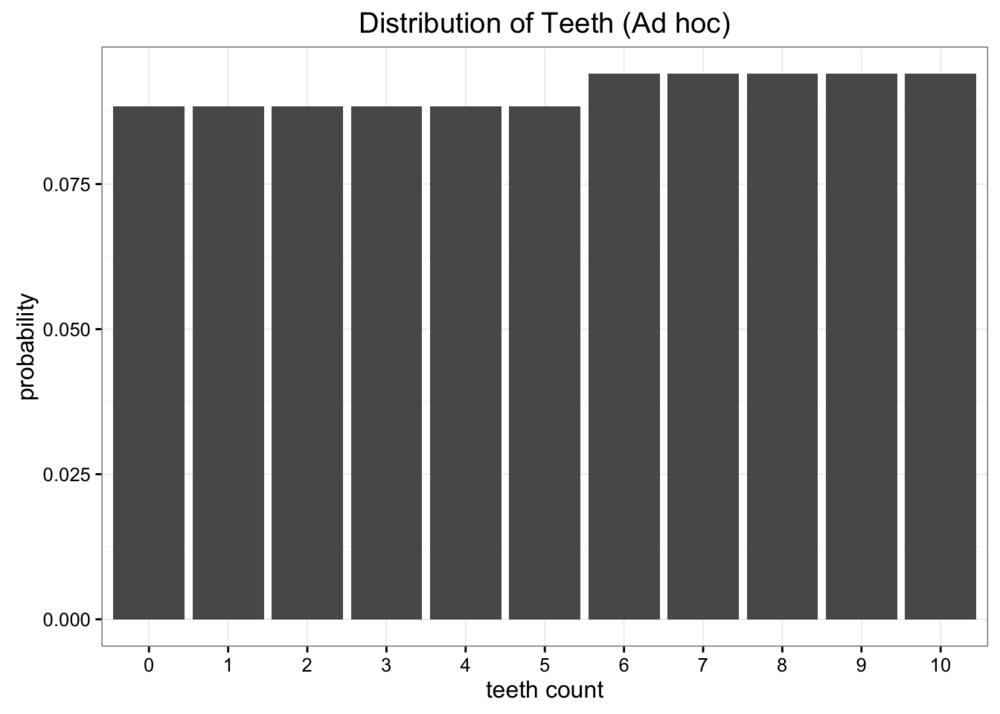Kullback-Leibler Divergence Explained 的翻译和笔记

# 笔记

KL散度原始分布中数据概率与近似分布中数据概率的对数差期望，用于量化参数化近似代替观测分布时丢失了多少信息。散度越小，说明模拟越逼近现实。

## 信息

熵作为度量信息的期望，引用PRML中的观点，信息量可以看成是在了解事物时，感受到的“惊讶程度”。低概率事件内蕴的信息会比高概率的信息多，比如革命性产品问世、突破性科学发现。正所谓“无常生妖”，低概率事件有更多信息符合直觉。

熵计算公式中的对数不大好理解，参考严谨推导可以发现信息量确实是$f(x)=-log{x}$。

$x_1$和$x_2$同时发生的概率：$P(x_1, x_2) = P(x_1)×P(x_2)$
$x_1$和$x_2$的总信息量：$log_2(P(x_1)P(x_2)) = log_2P(x_1)+log_2P(x_2)$

## 趣闻

H就是玻尔兹曼著名的H定理中的H

# 译文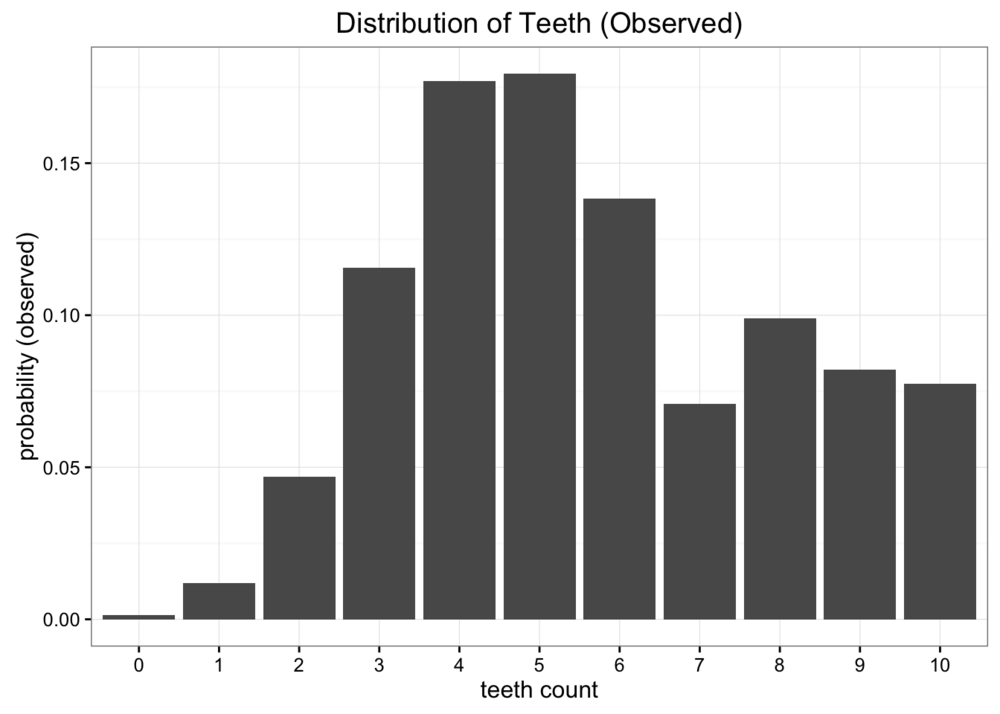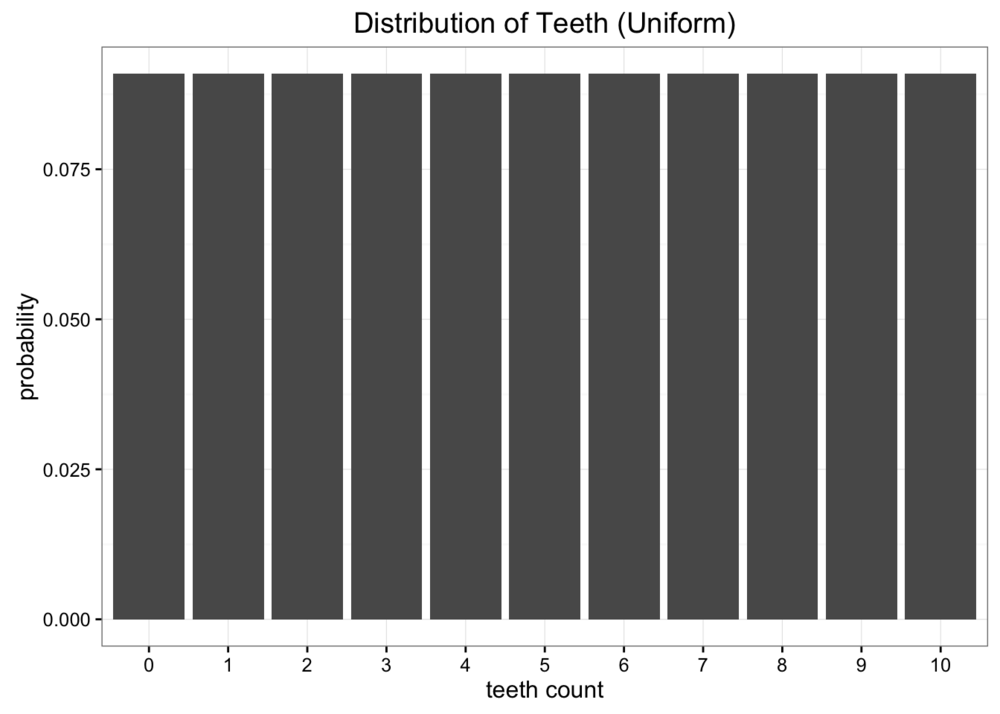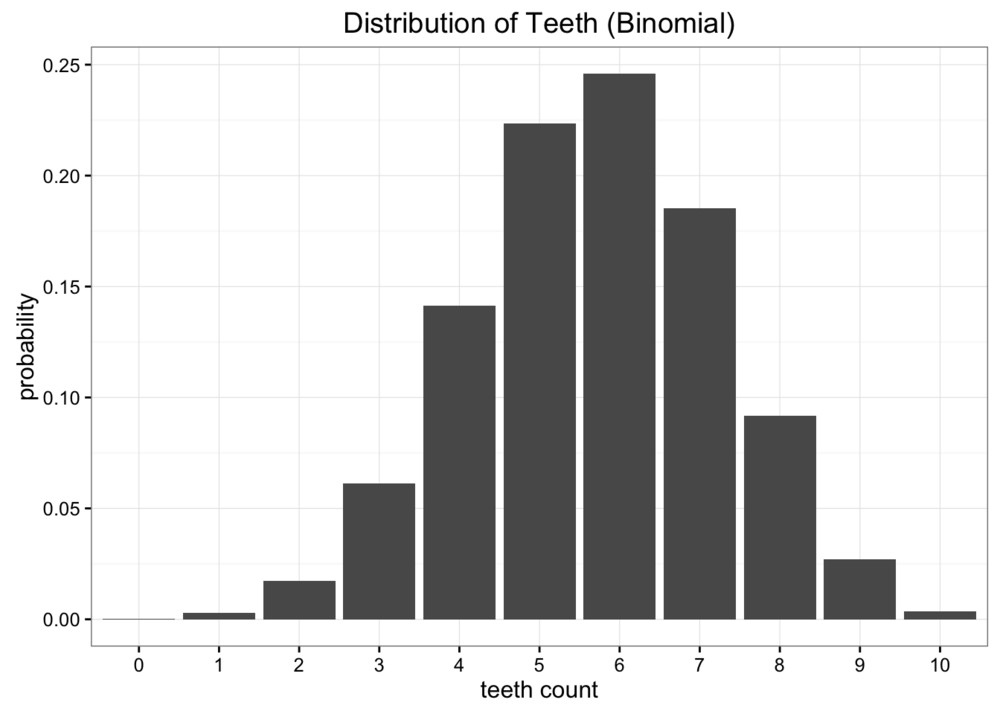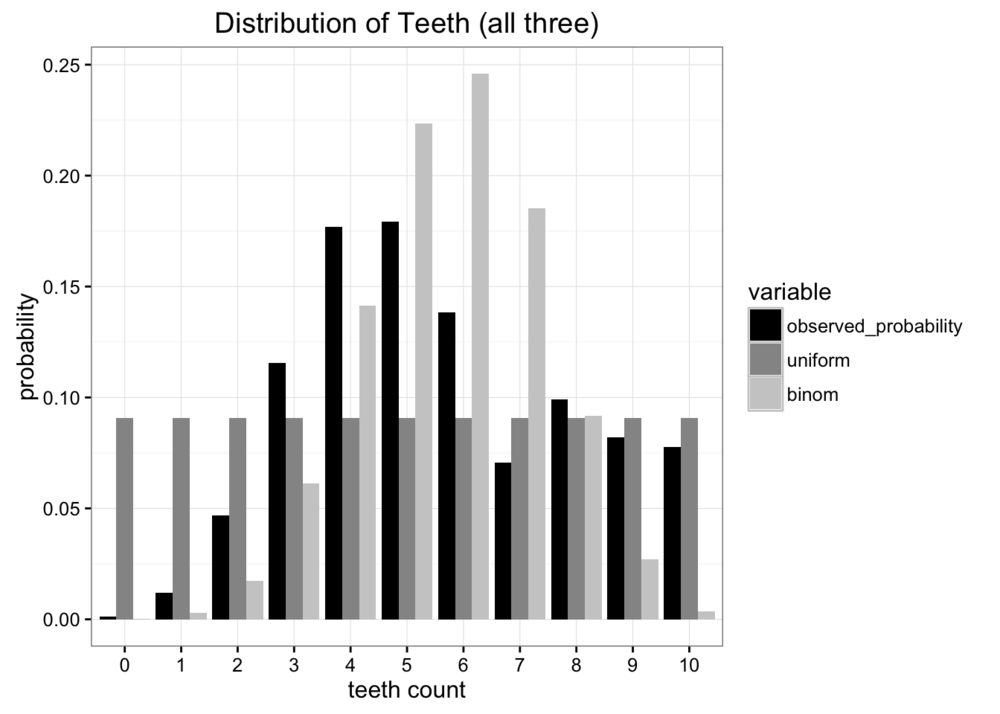## 分布的熵

KL散度起源于信息论。信息论的主要目标是量化数据中有多少信息。信息论中最重要的度量是熵，通常用$H$表示。概率分布的熵的定义是：

## 使用Kullback-Leibler散度度量丢失的信息

Kullback-Leibler散度只是对熵公式稍加修改。我们不仅用到概率分布 $p$，还加入了近似分布 $q$。然后我们看一下每一个对数值的差异:

KL散度更常见的写法如下:

## 使用KL散度进行优化